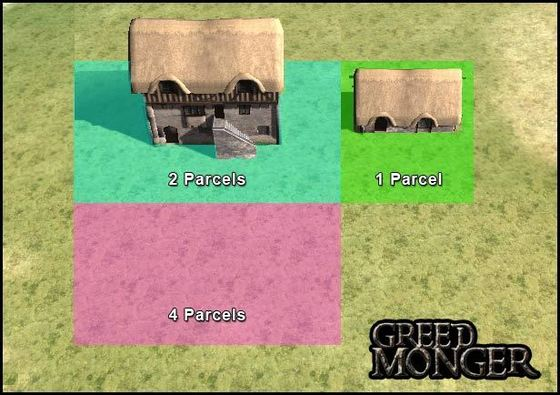# Parcel Size

#### Ronus

##### Member
JFF Member
Just an FYI, each parcel is 20 meters X 20 meters or 66ft X 66ft.

So the 4 parcel lot will be 131ft X 131ft, just a little more than 17,000 sq ft.

James Proctor said:
There was a mix up and Jason thought it was 3 meters = 1 unity unit... The Scaling we had talked about before was 1 unit = 1 meter. which would mean parcel sizes would be 20x20 meters. 1 unit equaling a meter makes sense in a lot of ways so the scaling will remain that way.
One of the Devs clarifying a remark that it was 60m X 60m.

Here is an example pic of the lot sizes: